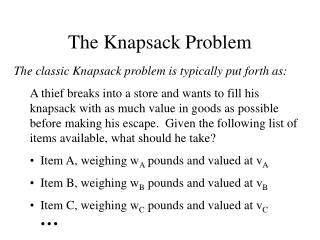DownloadDownload PresentationThe Knapsack Problem

# The Knapsack Problem

Download Presentation## The Knapsack Problem

- - - - - - - - - - - - - - - - - - - - - - - - - - - E N D - - - - - - - - - - - - - - - - - - - - - - - - - - -
##### Presentation Transcript

1. The Knapsack Problem • The classic Knapsack problem is typically put forth as: • A thief breaks into a store and wants to fill his knapsack with as much value in goods as possible before making his escape. Given the following list of items available, what should he take? • Item A, weighing wA pounds and valued at vA • Item B, weighing wB pounds and valued at vB • Item C, weighing wC pounds and valued at vC •   

2. The Knapsack Problem • Input • Capacity K • n items with weights wi and values vi • Goal • Output a set of items S such that • the sum of weights of items in S is at most K • and the sum of values of items in S is maximized

3. The Simplest Versions… • Can items be divided up such that only a portion is taken? • The thief can hold 5 pounds and has to choose from: • 3 pounds of gold dust at \$379.22/pound • 6 pounds of silver dust at \$188.89/pound • 1/9 pound of platinum dust at \$433.25/pound • Are all of the weights or total values identical? • The thief breaks into a ring shop where all of the rings weight 1oz. He can hold 12 ounces; which should he take?

4. A Deceptively Hard Version… What if each problem has the same price/pound? This problem reduces to the bin-packing problem: we want to fit as many pounds of material into the knapsack as possible. How can we approach this problem?

5. Example The thief breaks into a gold refinery; he can steal from a selection of raw gold nuggets, each of the same value per pound. If he can carry 50 pounds, what selection would maximize the amount he carries out? 47.3 pounds 6.0 pounds 5.2 pounds 36.7 pounds 5.6 pounds 5.2 pounds 25.5 pounds 5.6 pounds 5.0 pounds 16.7 pounds 5.4 pounds 3.2 pounds 8.8 pounds 5.3 pounds 0.25 pounds

6. An Easier Version... What if all of the sizes we are working with are relatively small integers? For example, if we could fit 10 pounds and: Object A is 2 pounds and worth \$40 Object B is 3 pounds and worth \$50 Object C is 1 pound and worth \$100 Object D is 5 pounds and worth \$95 Object E is 3 pounds and worth \$30 We can use dynamic programming!

7. Definining subproblems • Define P(i,w) to be the problem of choosing a set of objects from the first i objects that maximizes value subject to weight constraint of w. • V(i,w) is the value of this set of items • Original problem corresponds to V(n, K)

8. Recurrence Relation • V(i,w) = max (V(i-1,w-wi) + vi, V(i-1, w)) • A maximal solution for P(i,w) either • uses item i (first term in max) • or does NOT use item i (second term in max) • V(0,w) = 0 (no items to choose from) • V(i,0) = 0 (no weight allowed)

9. The solution... Items wA = 2 vA = \$40 wB = 3 vB = \$50 wC = 1 vC = \$100 wD = 5 vD = \$95 wE = 3 vE = \$30 Weight

10. Define P(i,w) to be the problem of choosing a set of objects from the first i objects that maximizes value subject to weight constraint of w. V(i,w) is the value of this set of items Original problem corresponds to V(n, W) Define P(i,k) to be … Define Subproblems Based on Value